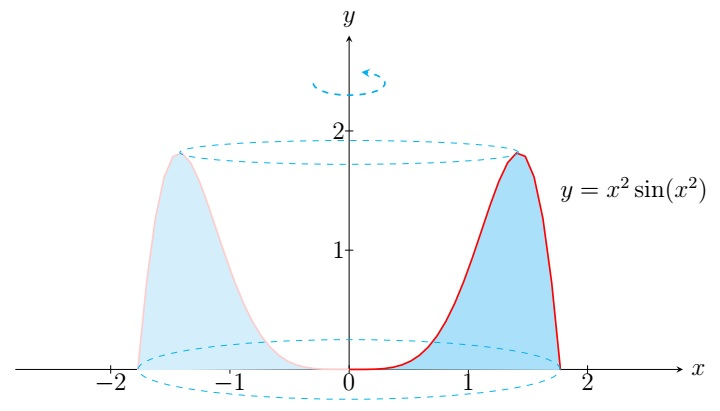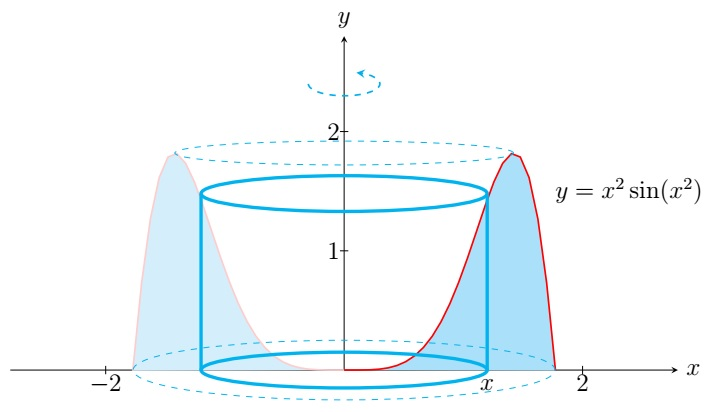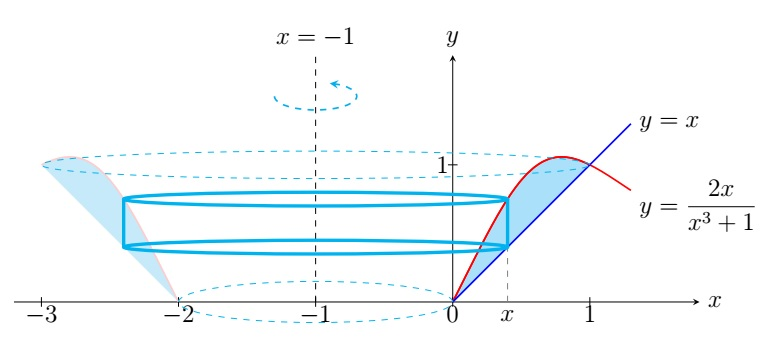Calculus II Home

## Cylindrical Shells Method

Consider the following problem:
Find the volume of the solid obtained by rotating the region bounded by $$y=x^2\sin(x^2)$$, $$x=0$$, $$x=\sqrt{\pi}$$, and $$y=0$$ about the $$y$$-axis.First note that the cross-section of the solid at $$y$$ perpendicular to the $$y$$-axis is a washer. To apply the washer method, we need to find the inner radius and the outer radius which will require to solve for $$x$$ in terms of $$y$$ from $$y=x^2\sin(x^2)$$. It is complicated and sometimes analytically impossible to solve for $$x$$ in terms of $$y$$ from $$y=f(x)$$. We have a better method for this kind of problems:

Cylindrical Shells Method:
Consider the region bounded by $$y=f(x)$$, $$x=a$$, $$x=b$$, and the $$x$$-axis. If $$S$$ is the solid obtained by rotating the region about the $$y$$-axis, then the volume of $$S$$ is $V(S)= \int_a^b 2\pi x f(x)\;dx.$ We can remember the preceding formula by associating $$2\pi x,\; f(x)$$, and $$dx$$ with the circumference, height, and thickness of the cylindrical shell with the radius $$x$$ respectively.

Break $$[a,b]$$ into $$n$$ subintervals $$[x_0,x_1],[x_1,x_2],\ldots,[x_{n-1},x_n]$$ where $$x_i=a+i \Delta x$$ and $$\Delta x=(b-a)/n$$. Partition $$S$$ into $$n$$ solids $$S_1,S_2,\ldots,S_n$$ by intersecting $$S$$ by the vertical planes at $$x_1,x_2,\ldots,x_{n-1}$$. Then the volume of $$S_i$$ is approximately that of the cylindrical shell with the inner radius $$x_{i-1}$$, the outer radius $$x_i$$, and height $$f(x_i^*)$$ where $$x_i^*=\frac{x_{i-1}+x_i}{2}$$, $$i=1,2,\ldots,n$$. Therefore \begin{align*} V(S_i) &\approx \pi x_i^2 f(x_i^*)-\pi x_{i-1}^2 f(x_i^*)\\ &=\pi f(x_i^*)\left( x_i^2 -x_{i-1}^2\right)\\ &=\pi f(x_i^*) (x_i+x_{i-1})(x_i-x_{i-1})\\ &=2\pi f(x_i^*) \frac{x_{i-1}+x_i}{2} (x_i-x_{i-1})\\ &=2\pi f(x_i^*) x_i^* \Delta x. \end{align*} Thus the volume of $$S$$ is $V(S)\approx \sum_{i=1}^n 2\pi x_i^* f(x_i^*) \Delta x.$ This approximation of $$V(S)$$ gets better as $$n\to \infty$$. Thus $V(S)=\lim_{n\to \infty} \sum_{i=1}^n 2\pi x_i^* f(x_i^*) \Delta x=\int_a^b 2\pi x f(x)\;dx.$

Example. Find the volume of the solid obtained by rotating the region bounded by $$y=x^2\sin(x^2)$$, $$x=0$$, $$x=\sqrt{\pi}$$, and $$y=0$$ about the $$y$$-axis.

Solution.The volume of the solid is \begin{align*} & \int_0^{\sqrt{\pi}} 2\pi x\cdot x^2\sin(x^2)\;dx \\ =\;& \pi\int_0^{\sqrt{\pi}} x^2\sin(x^2) \cdot 2x\;dx \\ =\;& \pi\int_0^{\pi} u\sin u\;du \;\;\left( \text{Let } u=x^2. \text{ Then } du=2x\;dx\right) \\ =\;& \pi \left.\left( -u\cos u +\sin u \right)\right\vert_0^{\pi} \;\;\left( \text{Integrating by parts} \right) \\ =\;& \pi \left( -\pi\cos \pi +\sin \pi \right) -\pi \left( -0\cos 0 +\sin 0 \right) \\ =\;& \pi^2. \end{align*}

For some problems, the formula of Cylindrical Shells Method $$V(S)= \int_a^b 2\pi x f(x)\;dx$$ is modified by replacing $$x$$ and $$f(x)$$ by the radius and the height of the cylindrical shell at $$x$$ respectively.

Example. Find the volume of the solid obtained by rotating the region bounded by $$y=x$$ and $$y=\frac{2x}{x^3+1}$$ about the line $$x=-1$$.

Solution.First note that $$y=x$$ and $$y=\frac{2x}{x^3+1}$$ intersect at $$x=0,1$$. The cylindrical shell at $$x$$ has radius $$x-(-1)$$ and height $$\frac{2x}{x^3+1}-x$$. The volume of the solid is \begin{align*} & \int_0^1 2\pi (x+1) \left( \frac{2x}{x^3+1} -x\right)\;dx \\ =\;& 2\pi\int_0^1 2\pi \left[-(x+1)x+(x+1)\frac{2x}{x^3+1} \right]\;dx\\ =\;& 2\pi\int_0^1 2\pi \left[-x^2-x+\frac{2x}{x^2-x+1} \right]\;dx\\ =\;& 2\pi\int_0^1 2\pi \left[-x^2-x+\frac{2x-1}{x^2-x+1} +\frac{1}{\left(x-\frac{1}{2}\right)^2+\left( \frac{\sqrt{3}}{2} \right)^2} \right]\;dx\\ =\;& 2\pi \left.\left( -\frac{x^3}{3}-\frac{x^2}{2}+\ln|x^2-x+1|+\frac{2}{\sqrt{3}}\tan^{-1}\left(\frac{x-\frac{1}{2}}{\frac{\sqrt{3}}{2}} \right) \right)\right\vert_0^1 \\ =\;& 2\pi\left( -\frac{5}{6}+\frac{4}{\sqrt{3}} \tan^{-1}\left( \frac{1}{\sqrt{3}} \right) \right)\\ =\;& 2\pi\left( -\frac{5}{6}+\frac{2\pi}{3\sqrt{3}} \right). \end{align*}

The roles of $$x$$ and $$y$$ in the Cylindrical Shells Method may be switched for some problems.

Example. Find the volume of the solid obtained by rotating the region bounded by $$x=y(3-e^y)$$ and the $$y$$-axis about the $$x$$-axis.

Solution. First note that at the points of intersection of $$x=y(3-e^y)$$ and $$x=0$$, we have $y(3-e^y)=0\implies y=0,\ln 3.$ The cylindrical shell at $$y$$ has radius $$y$$ and height $$y(3-e^y)$$. The volume of the solid is \begin{align*} & \int_0^{\ln 3} 2\pi y \cdot y(3-e^y) \;dy\\ =\;& 2\pi\int_0^{\ln 3} \left(3y^2-y^2e^y \right) \;dy\\ =\;& 2\pi \left.\left(y^3-e^y(y^2-2y+2) \right) \right\vert_0^{\ln 3} \;\;\left( \text{Integrating by parts} \right) \\ =\;& 2\pi \left((\ln 3)^3-3((\ln 3)^2-2\ln 3+2) \right)+4\pi\\ =\;& 2\pi \left((\ln 3)^3-3(\ln 3)^2+6\ln 3-4) \right). \end{align*}

Last edited